# How to Stack Data from Multiple Columns into One Column in Excel

In previous article, I have shown you the method to split data from one long column to multiple columns by VBA and Index function. This time if we want to stack data from multiple columns to one column, how can we do? Actually, we can still use VBA script or formula of Index function to make it come true. This article will introduce you the two methods. After reading the article below, you will find the two ways are verify simple and convenient to operate in excel.

For example, see the initial table below: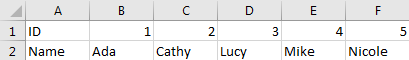And we want reverse data into one column and make it looks like: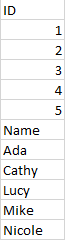Now we can follow below two methods to make it possible.

Method 1: Stack Data in Multiple Columns into One Column by Formula

Step 1: Select range A1 to F2 (you want to do stack), in Name Box, enter a valid name like Range, then click Enter.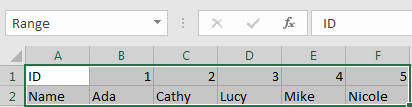Step 2: In any cell you want to locate the first cell of destination column, enter the formula =INDEX(Range,1+INT((ROW(A1)-1)/COLUMNS(Range)),MOD(ROW(A1)-1+COLUMNS(Range),COLUMNS(Range))+1).

Please be aware that you have to replace ‘Range’ in this formula to your defined name in Name Box.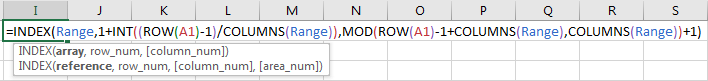Step 3: Click Enter. Verify that ‘ID’ (the value in the first cell of selected range) is displayed properly.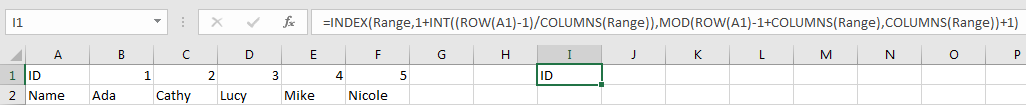Step 4: Drag the fill handle to fill I column. Verify that data in previous initial location is reversed to one column properly.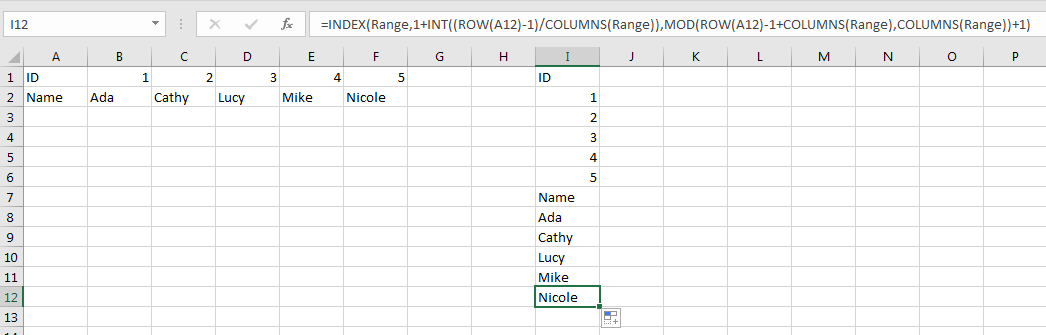Note:

If you drag the fill handle to cells extend selected range cell number, error will be displayed in redundant cell.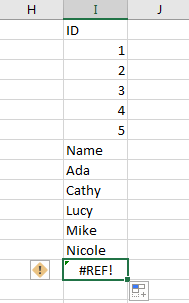## Method 2: Stack Data in Multiple Columns into One Column by VBA

Step 1: On current visible worksheet, right click on sheet name tab to load Sheet management menu. Select View Code, Microsoft Visual Basic for Applications window pops up.

Or you can enter Microsoft Visual Basic for Applications window via Developer->Visual Basic.

Step 2: In Microsoft Visual Basic for Applications window, click Insert->Module, enter below code in Module1:

```Sub StackDataToOneColumn()

Dim Rng1 As Range, Rng2 As Range, Rng As Range

Dim RowIndex As Integer

Set Rng1 = Application.Selection

Set Rng1 = Application.InputBox("Select Range:", "StackDataToOneColumn", Rng1.Address, Type:=8)

Set Rng2 = Application.InputBox("Destination Column:", "StackDataToOneColumn", Type:=8)

RowIndex = 0

Application.ScreenUpdating = False

For Each Rng In Rng1.Rows

Rng.Copy

Rng2.Offset(RowIndex, 0).PasteSpecial Paste:=xlPasteAll, Transpose:=True

RowIndex = RowIndex + Rng.Columns.Count

Next

Application.CutCopyMode = False

Application.ScreenUpdating = True

End Sub```

Step 3: Save the codes, see screenshot below. And then quit Microsoft Visual Basic for Applications.

Step 4: Click Developer->Macros to run Macro. Select ‘StackDataToOneColumn’ and click Run.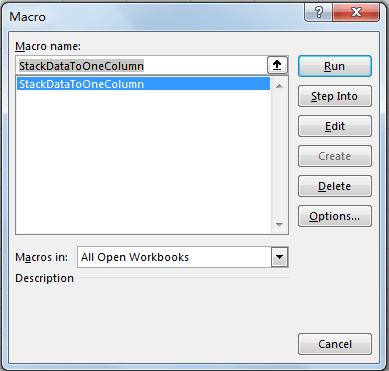Step 5: Stack Data to One Column dialog pops up. Enter Select Range \$A\$1:\$F\$2. Click OK. In this step you can select the range you want to do stack.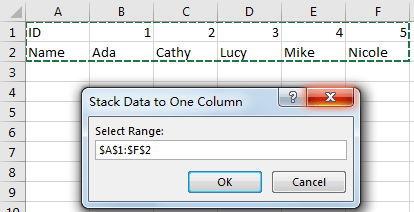Step 6: On Stack Data to One Column, enter Destination Column \$I\$1. Click OK. In this step you can select the first cell from destination range you want to save data.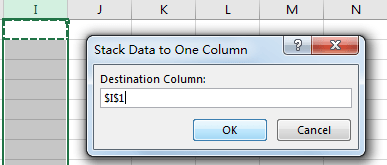Step 7: Click OK and check the result. Verify that data is displayed in one column properly. The behavior is as same as the result in method 1 step# 4.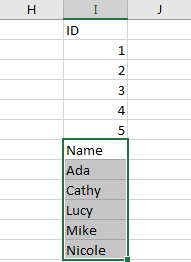### Related Functions

• Excel INDEX function
The Excel INDEX function returns a value from a table based on the index (row number and column number)The INDEX function is a build-in function in Microsoft Excel and it is categorized as a Lookup and Reference Function.The syntax of the INDEX function is as below:= INDEX (array, row_num,[column_num])…
• Excel ROW function
The Excel ROW function returns the row number of a cell reference.The ROW function is a build-in function in Microsoft Excel and it is categorized as a Lookup and Reference Function.The syntax of the ROW function is as below:= ROW ([reference])….
• Excel MOD function
he Excel MOD function returns the remainder of two numbers after division. So you can use the MOD function to get the remainder after a number is divided by a divisor in Excel. The syntax of the MOD function is as below:=MOD (number, divisor)….
• Excel INT function
The Excel INT function returns the integer portion of a given number. And it will rounds a given number down to the nearest integer.The syntax of the INT function is as below:= INT (number)…
• Excel COLUMNS function
The Excel COLUMNS function returns the number of columns in an Array or a reference.The syntax of the COLUMNS function is as below:=COLUMNS (array)….
Related Posts

VBA Macro For VLOOKUP From Another Sheet

In the previous post, you should know that how to fix or remove the #N/A error when using VLOOKUP formula to lookup value from another sheet. And this post will show you how to use VBA code to vlookup data ...

How To Insert Comments in Protected Worksheet in Excel

This post will show you how to allow comments in a protected worksheet in Excel. You can easily to insert comments into cells in a normal worksheet in Excel, but if want to insert a comment in a worksheet that ...

How To Convert Text to Upper Cases(Using VBA) in Excel

This post will show you how to switch from lower case to upper case in Excel. and I am going to show you two different ways of converting text to upper cases using formula or VBA macro in Excel 2013,Excel ...

How To Hide Every Other Row in Excel (Using VBA)

This post will show you how to hide alternate rows or columns in Excel or how to hide every third, fourth, fifth row or column in Excel. If you want to hide every other row in your current worksheet, how ...

How to Disable the Save As Prompt in Excel

This post will show you how to use a VBA Macro to save an Excel file and overwrite any existing file without a prompt so that you are going to get the little window that says file already exists do ...

How to Count Cells that Contain even or odd numbers in Excel

This post will guide you how to count the number of cells that contain odd or even numbers within a range of cells using a formula in Excel 2013/2016.How do I count cells that contain odd numbers through the use ...

How to Count Cells that Contain negative Numbers in Excel

This post will guide you how to count the number of cells that contain negative numbers within a range of cells using a formula in Excel 2013/2016.You can count the number of negative numbers in your data using easy functions ...

How to Count Cells Are Not Blank or Empty in Excel

This post will guide you how to count cells that are not blank or empty in a given range cells using a formula in Excel 2013/2016.How do I count the number of cells that are not blank in a particular ...

How to Count Cells Less Than a Specific Value in Excel

This post will guide you how to count the number of cells less than a particular numeric value in a given range cells using a formula in Excel 2013/2016. How do I count cells that are less than a specific ...

How to Count Cells Greater Than a Specific Value in Excel

This post will guide you how to count the number of cells greater than a particular numeric value in a given range cells using a formula in Excel 2013/2016. How do I count cells that are greater than a specific ...

Sidebar